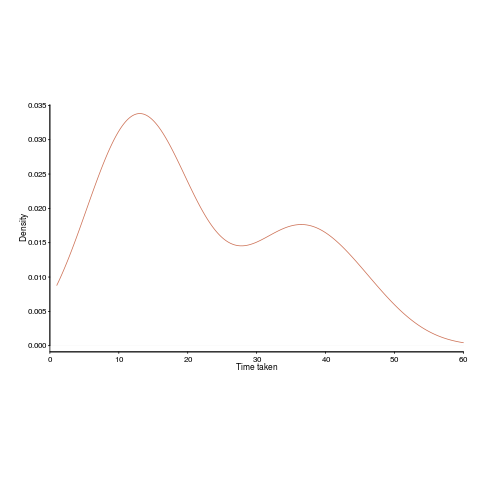## Some pair programming benefits may be mathematical artefacts

Many claims are made about the advantages of pair programming. The claim that the performance of pairs is better than the performance of individuals may actually be the result of the mathematical consequences of two people working together, rather than working independently (at least for some tasks).

Let’s say that individuals have to find a fault in code, and then fix it. Some people will find the fault and then its fix much more quickly than others. The data for the following analysis comes from the report Experimental results on software debugging (late Rome period), via Lutz Prechelt and shows the density of the time taken by each developer to find and fix a fault in a short Fortran program.

Fixing faults is different from many other development tasks in that if often requires a specific insight to spot the mistake; once found, the fixing task tends to be trivial.The mean time taken, for task t1, is 22.2 minutes (standard deviation 13).

How long might pairs of developers have taken to solve the same problem. We can take the existing data, create pairs, and estimate (based on individual developer time) how long the pair might take (code+data).

Averaging over every pair of 17 individuals would take too much compute time, so I used bootstrapping. Assuming the time taken by a pair was the shortest time taken by the two of them, when working individually, sampling without replacement produces a mean of 14.9 minutes (sd 1.4) (sampling with replacement is complicated…).

By switching to pairs we appear to have reduced the average time taken by 30%. However, the apparent saving is nothing more than the mathematical consequence of removing larger values from the sample.

The larger the variability of individuals, the larger the apparent saving from working in pairs.

When working as a pair, there will be some communication overhead (unless one is much faster and ignores the other developer), so the saving will be slightly less.

If the performance of a pair was the mean of their individual times, then pairing would not change the mean performance, compared to working alone. The performance of a pair has to be less than the mean of the performance of the two individuals, for pairs to show an improved performance.

There is an analytic solution for the distribution of the minimum of two values drawn from the same distribution. Ifis a probability density function andthe corresponding cumulative distribution function, then the corresponding functions for the minimum of a pair of values drawn from this distribution is given by:and.

The presence of two peaks in the above plot means the data is not going to be described by a single distribution. So, the above formula look interesting but are not useful (in this case).